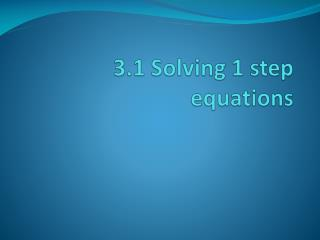# 3.1 Solving 1 step equations - PowerPoint PPT PresentationDownload Presentation3.1 Solving 1 step equations

3.1 Solving 1 step equationsDownload Presentation## 3.1 Solving 1 step equations

- - - - - - - - - - - - - - - - - - - - - - - - - - - E N D - - - - - - - - - - - - - - - - - - - - - - - - - - -
##### Presentation Transcript

1. 3.1 Solving 1 step equations

2. Notes • Equivalent: both sides of an equation are equal. • Ex: 2 + 2 = 4 • Inverse operation: operations that undoe each other. • Addition undoes subtr. • Subtr. Undoes addtion • State the inverse. Add 18 Subtract -25

3. State the inverse • Add -11 • Subtract –4.5 • Subtract 31 • Subtract -30.2

4. Notes • Linear equation • Variable raised to 1 • No power in denominator. • Nothing in square root • Not inside absolute value. • Linaear: • X + 5 = 9 • -4 + n = 2n – 6 • Nonlinear • X2 + 5 = 9 • X + 3 = 7

5. Solve Equation • X = 8 – 17 • X – (-19) = -9 • -15 = -13 + x • -11 = -2 + x

6. Solve equation • X + 6 = 10 • 5 - (-b) = 6 • -r – (-9) = 16

7. Word Problem • An airplane was at cruising altitude then descended 38o0 ft. If the plane is at 28,000 ft., what was the cruising altitude? • You hiked 8.3 mile in Denali National Park which is 2.7 miles farther than you hiked yesterday. How far did you hike yesterday?

8. Word Problem • Mt. McKinley is the highest point in the U.S. at 20,320ft. This is 8708 feet less than Mt. Everest. How high is Mt. Everest? • To convert celsius temp. to Kelvin temp. add 273.15 to the Celsius temp. What is -16 degrees celsius in Kelvin?

9. Wrap up • Questions/Comments • Ic: acuity “solving equations with addition and subtraction” • Hw: text pg. 135, #’s: 14-52 evens, 65